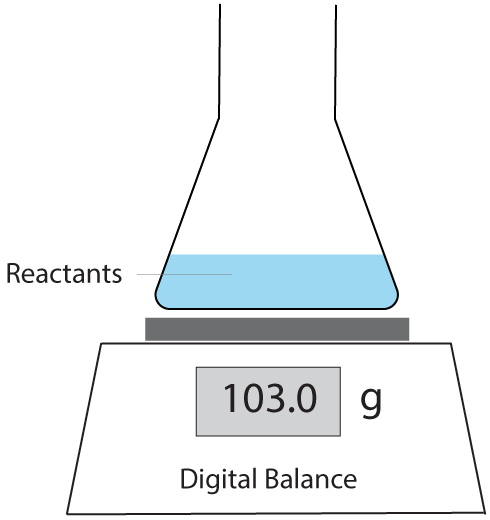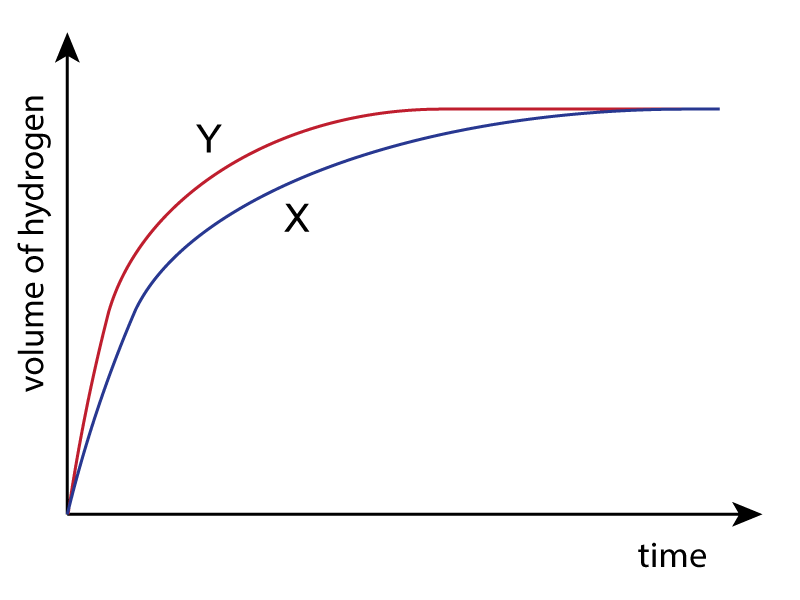Chemistry Edexcel iGCSE: Rates

Page 1

1. Which reaction between marble chips and acid would be fastest?
• A.   5g of powdered marble and hot acid
• B.   5g of powdered marble and cold acid
• C.   5g of marble chips and hot acid
• D.   5g of marble chips and cold acid

2. The diagram shows the apparatus used to measure the rate of a chemical reaction.For which of the following reactions can the rate be measured using this apparatus?

 I. NaOH  +  HCl  →  NaCl  +  H2O II. CaCO3  +  2HCl  →  CaCl2  +  H2O   +  CO2 III. 2H2O2  →  2 H2O   +  O2

• A.   all of them
• B.   I and II only
• C.   I and III only
• D.   II and III only
3. A student investigated the rate of reaction between zinc and excess sulfuric acid.

The graph shows the results of two experiments, X and Y.Which one of the following would explain the difference between X and Y?

• A.   Y was carried out at a higher temperature
• B.   Y was carried out using less concentrated acid
• D.   X was carried out using powdered zinc

4. Which changes decrease the rate of reaction between calcium carbonate and dilute hydrochloric acid?

 I. Decrease the temperature II. Decrease the concentration of acid III. Decrease the size of the calcium carbonate pieces

• A.   I, II and III
• B.   I and II only
• C.   I and III only
• D.   II and III only
5. Which of the following is NOT true about catalysts?
• A.   Catalysts are not chemically changed in the reaction
• B.   Catalysts speed up a reaction
• C.   Catalysts provide an alternative path of lower activation energy
• D.   Catalysts do not take part in the reaction

Q6-7:
A student adds small pieces of magnesium to dilute hydrochloric acid in a test tube. The reaction is exothermic.

6. What does the student see as the magnesium reacts and what happens to the temperature of the acid during the reaction?

 Observation Temperature A bubbles falls B bubbles rises C Solution turns cloudy falls D Solution turns cloudy rises
7. Two ways in which the student could make the reaction faster are..
• A.   Cool the acid and add water to the acid
• B.   Use warmer acid and one large piece of magnesium
• C.   Add water to the acid and powder the magnesium
• D.   Increase the acid concentration and use warmer acid
8. Oxygen is formed when manganese(IV)oxide is added to hydrogen peroxide, H2O2 in the following decomposition reaction:

2H2O2  →  2H2O   +   O2

The manganese(IV) oxide acts as …..

• A.   a reducing agent
• B.   a catalyst
• C.   an acid
• D.   a base

9. A 1.0 mol/dm3 solution of hydrochloric acid reacts more slowly with marble chips than the same volume of a 2.0 mol/dm3 solution.

Which of the following is the correct reason for this observation?

 1.0 mol/dm3 acid contains.. Collisions between reactants are.. A less particles per cm3 more frequent with 1.0 mol/dm3 acid B less particles per cm3 less frequent with 1.0 mol/dm3 acid C more particles per cm3 more frequent with 1.0 mol/dm3 acid D more particles per cm3 less frequent with 1.0 mol/dm3 acid

10. A student investigates the effect of four solids, A,B,C and D, on the decomposition of hydrogen peroxide using the following apparatus:He adds 1g of solid to 25cm3 of hydrogen peroxide solution and records the time taken to collect 20cm3 of oxygen. He then filters off, dries and weighs the solid.
Which solid acted as the best catalyst according to the following results?

 Time taken to collect 20cm3 oxygen Mass of solid after experiment A 106s 0.8g B 58s 1g C 9s 0.7g D 24s 1g